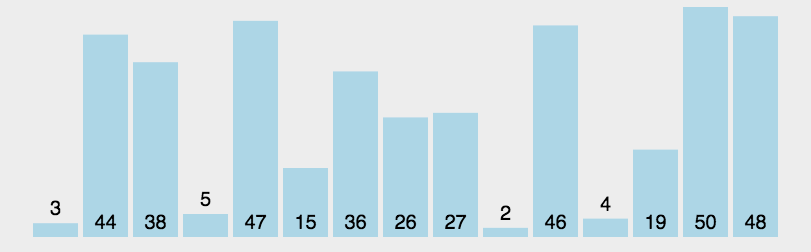# （2）快速排序 (Quick Sort)

2021-01-09 14:29 更新

• 从数列中挑出一个元素，称为 “基准”（pivot）；
• 重新排序数列，所有元素比基准值小的摆放在基准前面，所有元素比基准值大的摆在基准的后面（相同的数可以到任一边）。在这个分区退出之后，该基准就处于数列的中间位置。这个称为分区（partition）操作；
• 递归地把小于基准值元素的子数列和大于基准值元素的子数列排序。

### 动图演示### 代码实现

``````class Solution:
def partition(self, nums, begin, end):
pivot, counter = end, begin
# counter 记录小于pivot的位置和个数
for i in range(begin, end):
if nums[i] < nums[pivot]:
counter += 1
if counter != i:
temp = nums[counter]
nums[counter] = nums[i]
nums[i] = temp

temp = nums[pivot]
nums[pivot] = nums[counter]
nums[counter] = temp
return counter

def quicksort(self, nums, begin, end):
if end <= begin: return
pivot = self.partition(nums, begin, end)
self.quicksort(nums, begin, pivot - 1)
self.quicksort(nums, pivot + 1, end)

def sortArray(self, nums: List[int]) -> List[int]:
self.quicksort(nums, 0, len(nums) - 1)
return nums``````

#### 算法特性时间复杂度（最好）：O ( n l o g n ) O(nlog n)O(nlogn)时间复杂度（最坏）：O ( n 2 ) O(n^2)O(n2)时间复杂度（平均）：O ( n l o g n ) O(nlog n)O(nlogn)空间复杂度：O ( n l o g n ) O(nlog n)O(nlogn)稳定性：不稳定

App下载